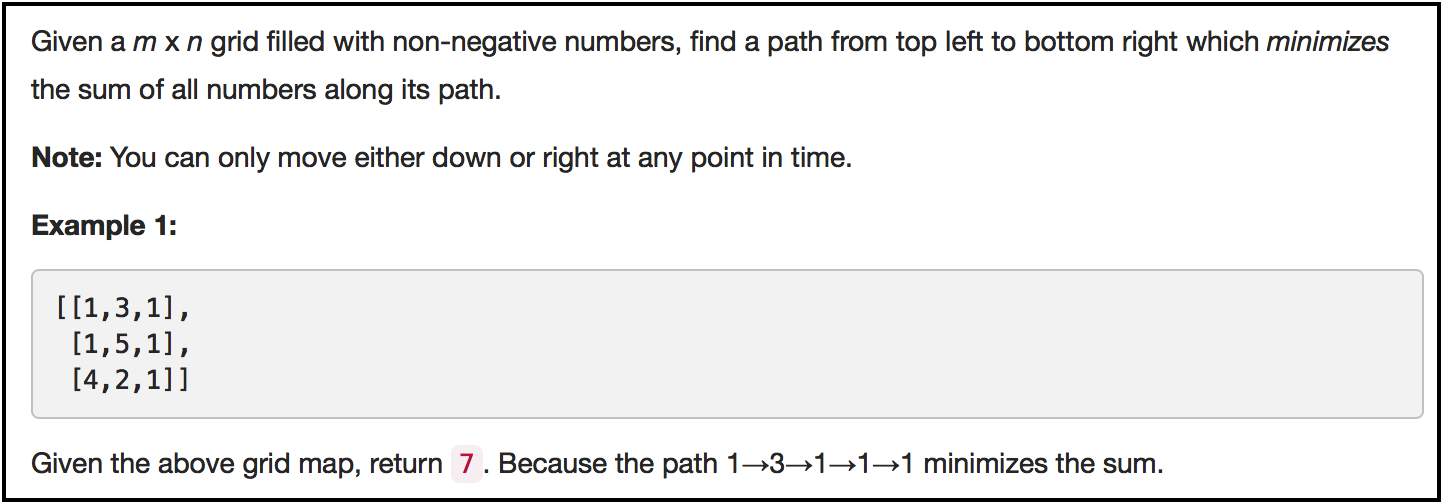# LeetCode中的动态规划题目解答（2）class Solution {
public:

int recursivePath(int x, int y, vector<vector<int>>& grid){

if(x==0 && y==0)
return grid;

if(x==0)
return recursivePath(x, y-1, grid)+grid[x][y];

if(y==0)
return recursivePath(x-1, y, grid)+grid[x][y];

int min_value = recursivePath(x-1, y, grid)<recursivePath(x, y-1, grid)?
recursivePath(x-1, y, grid):recursivePath(x, y-1, grid);

return min_value+grid[x][y];

}

int minPathSum(vector<vector<int>>& grid) {

int m = grid.size();
if(m==0) return 0;
int n = grid.size();
if(n==0) return 0;

return recursivePath(m-1, n-1, grid);
}
};

class Solution {
public:

int ** matrix;

int recursivePath(int x, int y, vector<vector<int>>& grid){

if (matrix[x][y] != -1)
return matrix[x][y];

if(x==0)
{
matrix[x][y] = recursivePath(x, y-1, grid)+grid[x][y];
return matrix[x][y];
}

if(y==0)
{
matrix[x][y] = recursivePath(x-1, y, grid)+grid[x][y];
return matrix[x][y];
}

matrix[x][y] = (recursivePath(x-1, y, grid)<recursivePath(x, y-1, grid)?
recursivePath(x-1, y, grid):recursivePath(x, y-1, grid))+grid[x][y];

return matrix[x][y];

}

int minPathSum(vector<vector<int>>& grid) {

int m = grid.size();
if(m==0) return 0;
int n = grid.size();
if(n==0) return 0;

matrix = new int*[m];

for(int i = 0; i < m; i++)
{
matrix[i] = new int[n];
for(int j = 0; j < n; j++)
{
matrix[i][j] = -1;
}
}

matrix = grid;

return recursivePath(m-1, n-1, grid);
}
};

（本文完）

• # 逆波兰表达式（Reverse Polish Notation）（#150）

©️2019 CSDN 皮肤主题: 精致技术 设计师: CSDN官方博客